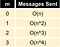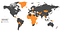# Understanding Blockchain Fundamentals, Part 2: Proof of Work & Proof of Stake

## Articles in this Understanding Blockchain Fundamentals series:Running time of solving the Byzantine Generals Problem with the algorithm proposed by Lamport, Shostak and Pease (n = number of actors, m = number of traitors)

Blockchains use consensus algorithms to elect a leader who will decide the contents of the next block.

# Proof of Work (PoW)

`Example:keccak256("hello") = 1c8aff950685c2ed4bc3174f3472287b56d9517b9c948127319a09a7a36deac8keccak256("hello1") = 57c65f1718e8297f4048beff2419e134656b7a856872b27ad77846e395f13ffe`
`Example - hash is a hypothetical hash function that has the outputs listed as belowY = 10, X = 'test'hash(X) = hash('test') = 0x0f = 15 > 10hash(X+1) = hash('test1') = 0xff = 255 > 10 hash(X+2) = hash('test2') = 0x09 = 9 < 10 OK, Solved.`Almost all African countries (separately) consume less electricity than the Bitcoin Mining industry

# Conclusion

Written by

Written by

## Georgios Konstantopoulos

#### Research @paradigm. prev: independent consultant